Search

Determinants: 3×3 Determinants (page 2 of 2)

Sections: 2×2 determinants, 3×3 determinantsThe computations for 3×3 determinants are messier than for 2×2's. Various methods can be used, but the simplest is probably the following:   Copyright © Elizabeth Stapel 2004-2011 All Rights Reserved

 Take a matrix A: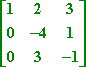Write down its determinant: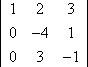Extend the determinant's grid by rewriting the first two columns of numbers: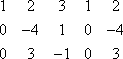Then multiply along the down-diagonals: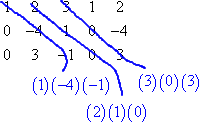...and along the up-diagonals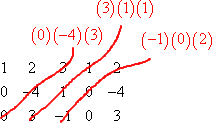Add the down-diagonals and subtract the up-diagonals: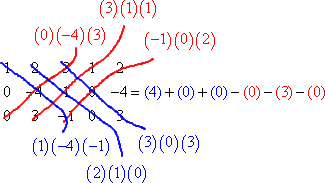And simplify: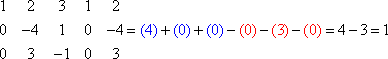Then det(A) = 1.

• Find the deteriminant of the following matrix:
•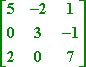First I convert from the matrix to its determinant, with the extra columns: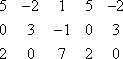Then I multiply down and up the diagonals: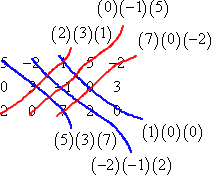Then I add the down-diagonals, subtract the up-diagonals, and simplify for the final answer: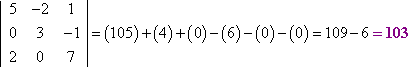There are other methods for simplifying determinants by hand, and these other methods are required when evaluating larger determinants by hand, but those methods can probably wait until later. For the time being, note that your graphing calculator should be able to evaluate the determinant of any (square) matrix you enter. For instance: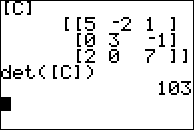But make sure, even if you have a graphing calculator, that you can evaluate 2×2 and 3×3 determinants, because you are likely to have word problems where the determinants contain variables that your calculator can't handle.

<< Previous  Top  |  1 | 2  |  Return to Index

 Cite this article as: Stapel, Elizabeth. "Determinants: 3x3 Determinants." Purplemath. Available from     https://www.purplemath.com/modules/determs2.htm. Accessed [Date] [Month] 2016

Study Skills Survey

Tutoring from Purplemath
Find a local math tutor

 Copyright © 2021  Elizabeth Stapel   |   About   |   Terms of Use   |   Linking   |   Site Licensing Contact Us